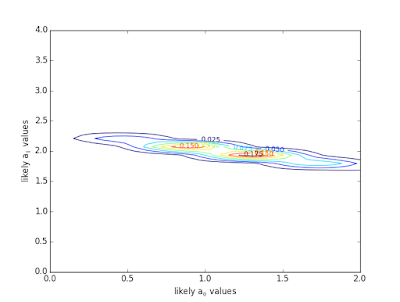## Monday, June 29, 2015

### Phase II.

Now that we understood how to simulate data measured with random noise, it was time to find the best fitting parameters for the data.  As an example, we used data that represented a linear trend.  We also specified 5 x-axis points, which implies that we have 5 data points each with a y-axis value called "d" (for data).
If you want to follow along, feel free to download (with the Terminal "wget" command after clicking RAW) or copy and paste my Python script into another script.Figure 1: Five data points representing 5 measurements with uncertainties associated with them.  To create this plot, I only needed an array of x-axis points and the two coefficients (a) for the linear equation: $$a_0 + a_1\times x_{vals}$$ .   There are uncertainties for each measurement due to what we, in the Astro-Statistics lecture, call "noise."  This random noise is added to the linear equation to simulate realistic measurements.  This plot was generated by calling my "poly" function.  For example, >>>execfile('stat_ex')       >>>poly(x_vals, a_vals, sigmas, plot=True)
As Figure 1 implies, a satisfactory model to fit that data would more than likely be a linear equation.  Figure 1 was forged by actually knowing the correct fitting parameters from the beginning: $$a_0 = 1$$ and $$a_1 = 2$$ .  The goal of this exercise is to use the simulated $$d_{vals}$$, i.e. measurements, to figure out two a coefficients that would provide a satisfactory linear model to fit the data.

The probability function will allow me to find satisfactory fitting parameters:
$P(d_1 | \mu ; \sigma_1 ) = \frac{1}{\sigma_1 \sqrt{2\pi} } e^{-\frac{1}{2} \Big(\frac{d_1 - \mu}{\sigma_1} \Big)^2 }$
P is the probability of me measuring $$d_1$$, which is dependent on the function $$\mu$$ and the uncertainty $$\sigma_1$$ of that specific measurement.  With the probability formula, I can find the maximum Likelihood and optimal $$\chi^2$$.  The derivation is as follows, where L is Likelihood:
$L = \prod_i P(d_i | \mu ; \sigma_i)$
$ln(L) = \sum_i ln\big[P(d_i | \mu ; \sigma_i )\big]$
$ln(L) = - \sum_i ln\big(\sigma_i \sqrt{2 \pi}) - \frac{1}{2}\sum_i \big(\frac{d_i - \mu}{\sigma_i}\big)^2$
$ln(L) = C - \frac{1}{2}\sum_i \chi^2$

Likelihood is the probability of measuring all of the data ("d_vals") that I measured.  This is why the probability of measuring each data point are multiplied together in the $$\Pi$$ symbol.  The C means that the first term is a mere constant, or offset, while the second term contains the famous $$\chi^2$$ expression.

Understanding how to use the equations is one thing.  Figuring out how to transfer that math language into a coding language is another thing entirely.  The example that I am about to convey is but one of many possible outcomes when running my Python script. If you repeatedly run the "poly" function in the command line, you will get different results and plots each time.  The following plots are exemplary of what you may see.

>>>execfile('stat_ex')
>>>poly(x_vals, a_vals, sigmas, plot=True)  # feel free to run this as many times as you wishFigure 2: Contour plot of the Likelihood matrix in my Python script.  The darker and redder regions represent where the Likelihood was the most.  Therefore, within that region lies the x and y coordinate pair that indicates the best fitting parameters: $$a_0$$ and $$a_1$$.  In this specific example, the contour suggests that the best fitting parameters are where $$a_1$$ is a little below 2.0 and $$a_0$$ ~ 1.40 .

The last two figures reiterate the results of Figure 2 but with much more clarity.  The matrix that was utilized to make the contour plot has now been transformed into a 1-dimensional version of the results.  These last figures confirm what was conjectured due to Figure 2.  The maximum Likelihood is found when $$a_0$$ is ~ 1.40 and $$a_1$$ is a tiny amount below 2.0.  The true values were $$a_0 = 1.0$$ and $$a_1 = 2.0$$.

This was a superb exercise for my first week of learning Astro-Statistics.